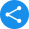# NCERT Class 5 Maths Book PDFView Notes

NCERT is an autonomous organisation, responsible for supplying school books to the students studying under CBSE. They print and publish books for every subject from class 1 to 12. NCERT books for class 5 maths is one of the books, which is hugely popular because of its innovative take on mathematics.

If you are a student of Class 5 CBSE, you must download class 5 maths NCERT book PDF as it covers all the concepts of your syllabus.

Apart from that, class 5 NCERT book maths also aids in enhancing the basic ideas of mathematics so that every student can learn these lessons with much enthusiasm.

 Class 5th Board CBSE Book NCERT Subject Maths Medium English / Hindi Study Materials Free Study Materials Available PDF Solutions Available in PDF Part 1 NCERT Books Class 5 Maths PDF Download

We have curated solutions for the books that you follow which you can access from the below links.

### NCERT Solutions for Class 5

1. What are the Topics Covered in Class 5 Mathematics?

Ans. There are a total of 14 chapters in class 5 mathematics that covers diverse topics like shapes and figures, patterns, measurements, mapping, charts, multiplication and divisions, etc. each chapter further contains quizzes and puzzles for making the book student-friendly.

2. How Can I Get Full Marks in Mathematics?

Ans. Getting full marks in mathematics is not an impossible task. For that, firstly, you need to understand the fundamentals of any topic. Then thorough practise and revision is the key to secure excellent grades. Additionally, you can follow NCERT books for class 5 maths and solve the exercise to ensure full marks in mathematics.

3. How Many Shapes are there in Class 5 Maths Syllabus? Which Figure has the Longest Perimeter?

Ans. Total 12 shapes are there in class 5 mathematics syllabus. Moreover, you can draw these all 12 figures using only 5 squares. For example, you can draw one or two squares and carve out another figure from that. Please refer to the textbook for a clear understanding.

A rectangle has the longest perimeter. More specifically, the perimeter of the “thinnest rectangle” is the least while it is the largest for “square-like” rectangle.

4. Which Shape Possesses the Most Area?

Ans. Among two 2-dimensional objects having the same perimeter, the circle possesses the most area. Similarly, among the polygons, a circular polygon has the most area. If you want to confirm this fact, then find the area using an appropriate formula like for circle, 2pir2

Share this with your friendsShare